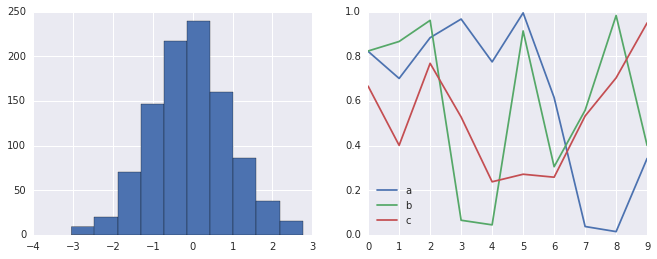# 8.14 自定义 Matplotlib：配置和样式表

Matplotlib 的默认绘图设置通常是其用户所抱怨的主题。虽然在 2016 年末的 Matplotlib 2.0 版本中有很多改进的内容，但自定义默认设置的能力，有助于使软件包符合你自己的审美偏好。

## 手动的绘图自定义

``````import matplotlib.pyplot as plt
plt.style.use('classic')
import numpy as np

%matplotlib inline

x = np.random.randn(1000)
plt.hist(x);
``````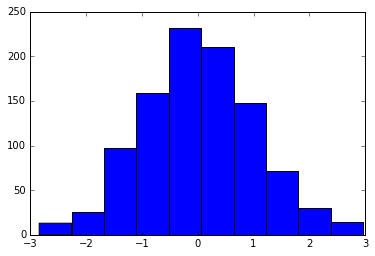``````# 使用灰色背景
ax = plt.axes(axisbg='#E6E6E6')
ax.set_axisbelow(True)

# 绘制白色实网格线draw solid white grid lines
plt.grid(color='w', linestyle='solid')

# 隐藏轴的刻度
for spine in ax.spines.values():
spine.set_visible(False)

# 隐藏顶部和右侧刻度
ax.xaxis.tick_bottom()
ax.yaxis.tick_left()

# 将刻度和标签变亮
ax.tick_params(colors='gray', direction='out')
for tick in ax.get_xticklabels():
tick.set_color('gray')
for tick in ax.get_yticklabels():
tick.set_color('gray')

# 控制直方图的人脸和边界颜色
ax.hist(x, edgecolor='#E6E6E6', color='#EE6666');
``````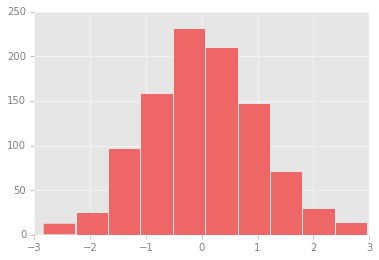## 修改默认值：`rcParams`

``````IPython_default = plt.rcParams.copy()
``````

``````from matplotlib import cycler
colors = cycler('color',
['#EE6666', '#3388BB', '#9988DD',
'#EECC55', '#88BB44', '#FFBBBB'])
plt.rc('axes', facecolor='#E6E6E6', edgecolor='none',
axisbelow=True, grid=True, prop_cycle=colors)
plt.rc('grid', color='w', linestyle='solid')
plt.rc('xtick', direction='out', color='gray')
plt.rc('ytick', direction='out', color='gray')
plt.rc('patch', edgecolor='#E6E6E6')
plt.rc('lines', linewidth=2)
``````

``````plt.hist(x);
``````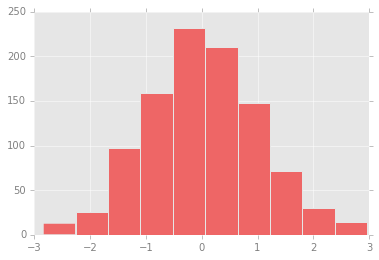``````for i in range(4):
plt.plot(np.random.rand(10))
``````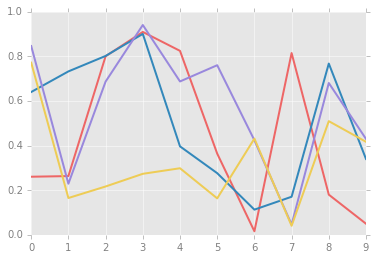## 样式表

2014 年 8 月发布的 Matplotlib 1.4 版增加了一个非常方便的`style`模块，其中包含许多新的默认样式表，以及创建和打包自己的样式的功能。这些样式表的格式与前面提到的`.matplotlibrc`文件类似，但必须使用`.mplstyle`扩展名来命名。

``````plt.style.available[:5]

'''
['fivethirtyeight',
'seaborn-pastel',
'seaborn-whitegrid',
'ggplot',
'grayscale']
'''
``````

``````plt.style.use('stylename')
``````

``````with plt.style.context('stylename'):
make_a_plot()
``````

``````def hist_and_lines():
np.random.seed(0)
fig, ax = plt.subplots(1, 2, figsize=(11, 4))
ax.hist(np.random.randn(1000))
for i in range(3):
ax.plot(np.random.rand(10))
ax.legend(['a', 'b', 'c'], loc='lower left')
``````

### 默认样式

``````# 重置 rcParams
plt.rcParams.update(IPython_default);
``````

``````hist_and_lines()
``````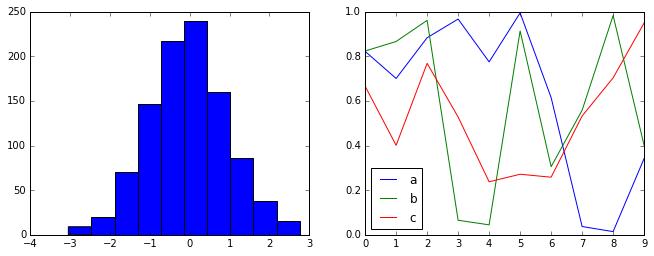### FiveThiryEight 样式

`fivethirtyeight`风格模仿流行的 FiveThirtyEight 网站上的图形。正如你在这里看到的那样，它以深色，粗线条和透明的轴为代表：

``````with plt.style.context('fivethirtyeight'):
hist_and_lines()
``````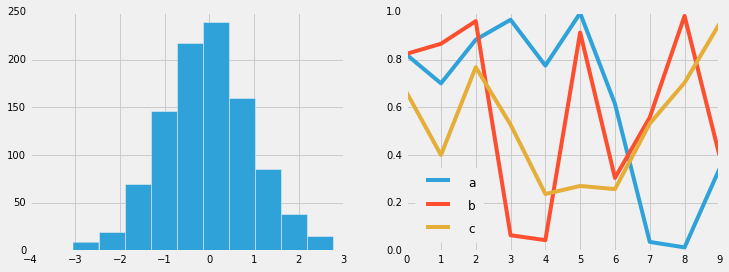### `ggplot`

R 语言中的`ggplot`包是非常流行的可视化工具。Matplotlib 的`ggplot`样式模仿该包的默认样式：

``````with plt.style.context('ggplot'):
hist_and_lines()
``````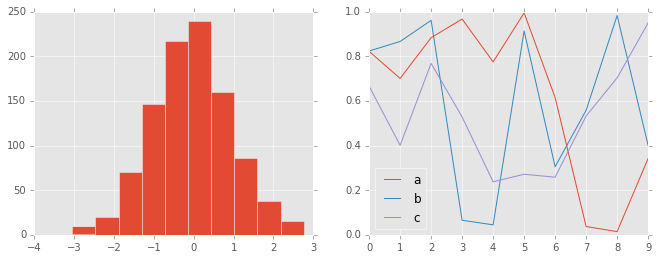### “黑客的贝叶斯方法”风格

``````with plt.style.context('bmh'):
hist_and_lines()
``````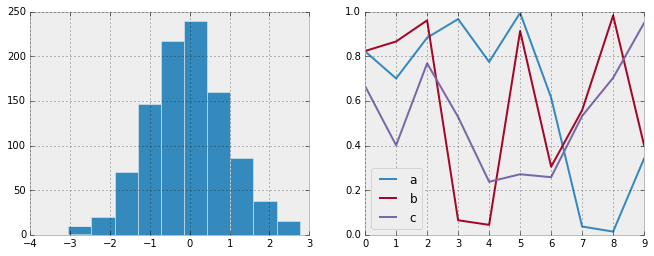### 暗黑背景

``````with plt.style.context('dark_background'):
hist_and_lines()
``````### 灰度

``````with plt.style.context('grayscale'):
hist_and_lines()
``````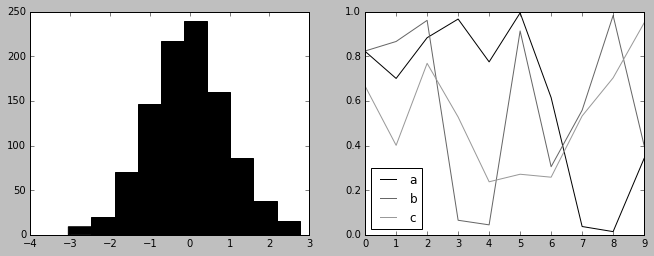### Seaborn 样式

Matplotlib 还有受 Seaborn 库启发的样式表（在“可视化和 Seaborn”中进行了更全面的讨论）。正如我们将看到的，将 Seaborn 导入笔记本时，这些样式会自动加载。我发现这些设置非常好，并且倾向于在我自己的数据探索中将它们用作默认设置。

``````import seaborn
hist_and_lines()
``````